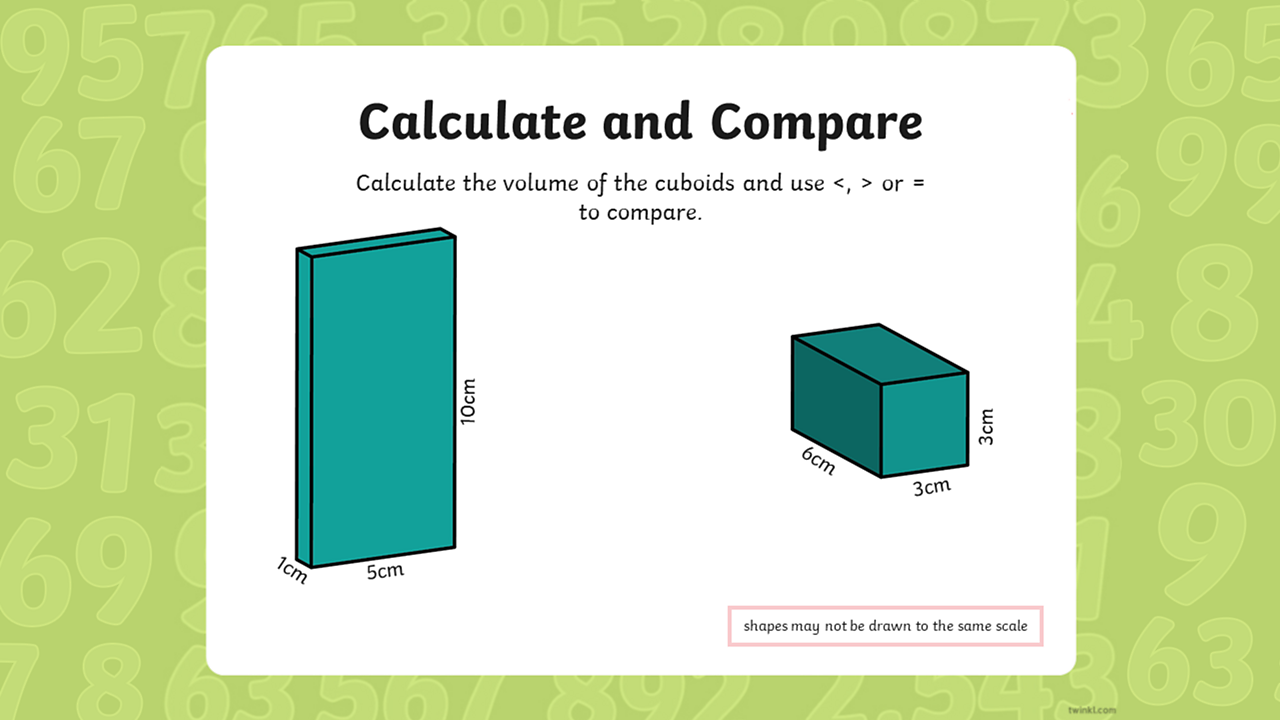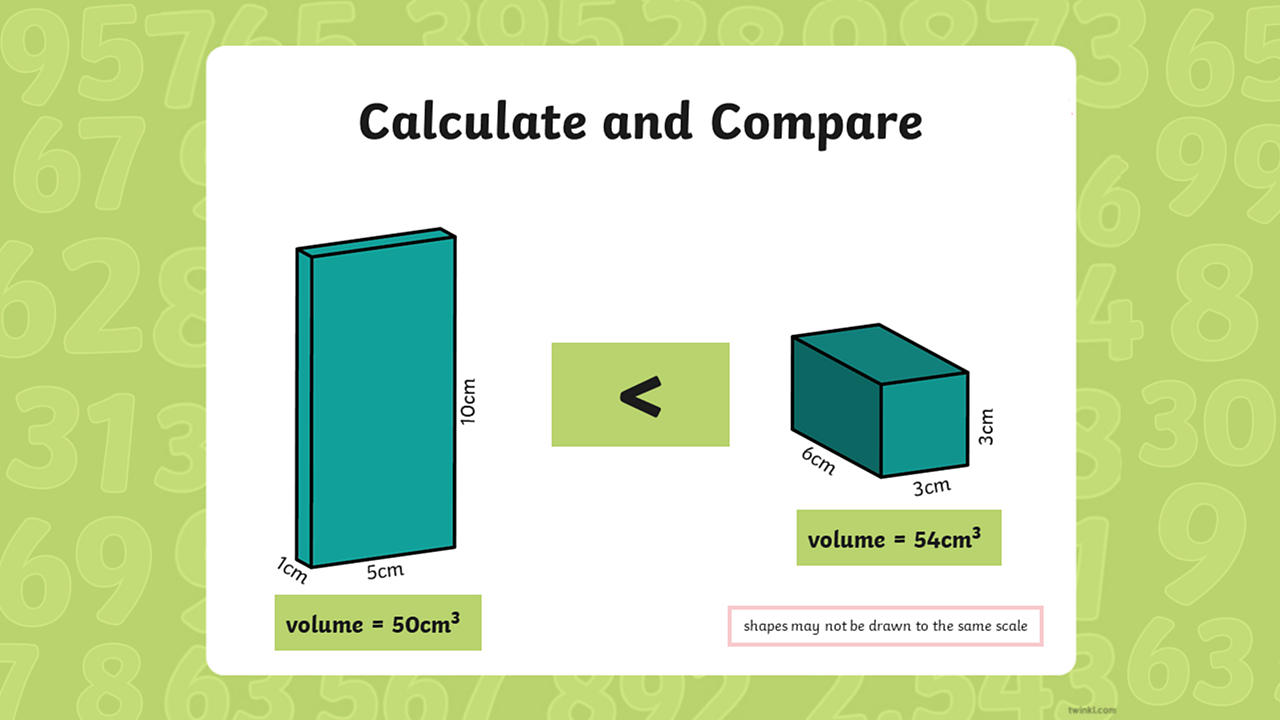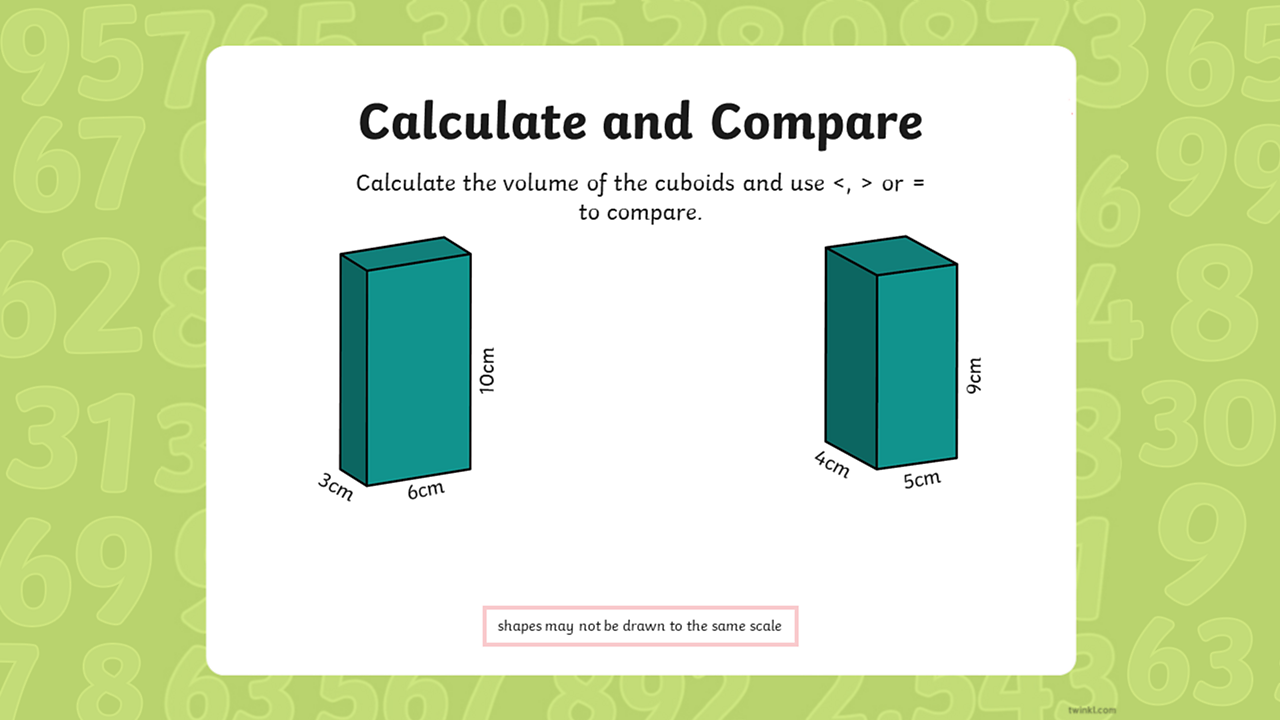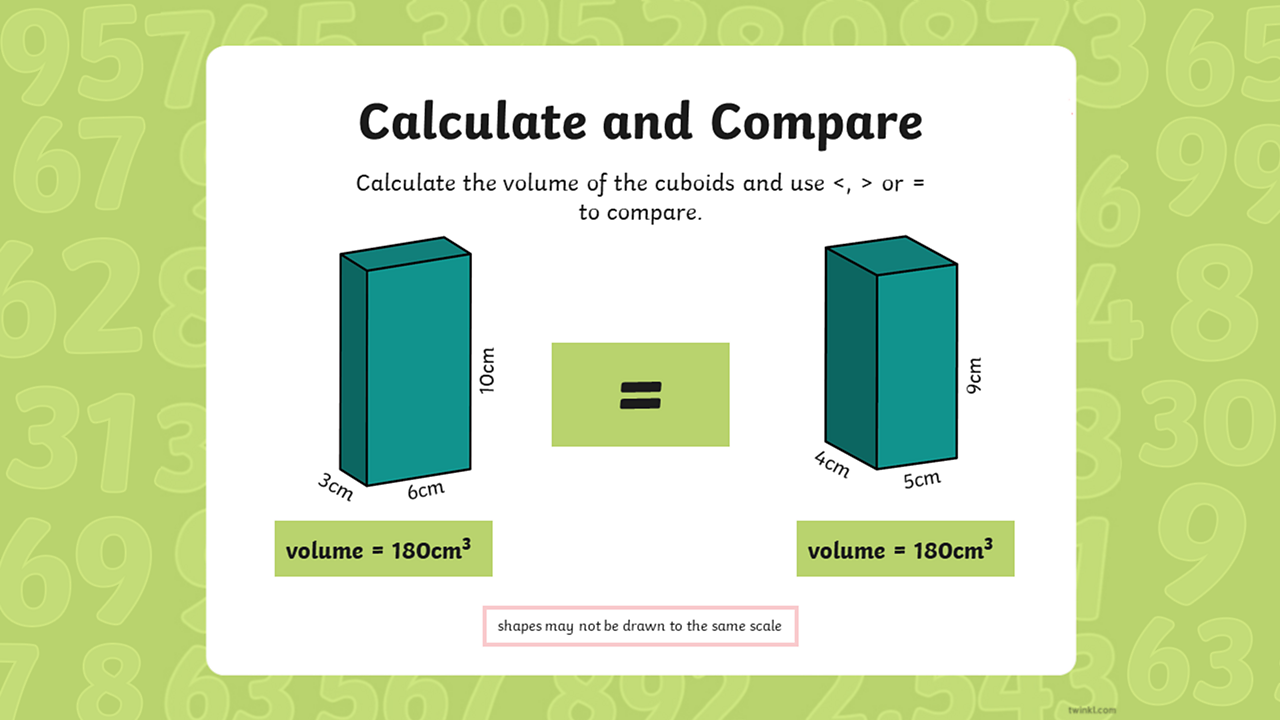# Calculating the volume of cubes and cuboids

## Home learning focus

Learn how to calculate, estimate and compare the volume of cubes and cuboids.

This lesson includes:

• two slideshows

Created in partnership with Twinkl

# Learn

In today's lesson, you will learn to calculate the volume of cubes and cuboids. To do so, we'll need to use the correct unit of measurement for finding the volume of fixed of a fixed object, called cubic units.

So today, we'll be using cubic centimetres (cm³) and cubic metres (m³) to measure the the volume of the cubes and cuboids. Find out more in the slideshows below.

You'll need something to jot down your answers as you work through the slideshows.

Now we know how to work out the volume of a cuboid, the next slideshow will explain how to calculate and compare the volume of cuboids.

Remember that:

• < means less than.
• > means greater thanFind the volume of these two shapes and then compare them. Which shape has the greater volume? Remember to use the formula length x width x height to workout the volumes.1 of 4Did you manage to compare the cuboids correctly?2 of 4Have a go at comparing the volume of these cuboids. You will need to calculate the volume of each shape first (L x H x W)3 of 4Both cuboids have the same volume. Can you think of two different cuboids that have the same volume?4 of 4

# Practise

## Activity 1

Find the volume of cubes and cuboids before attempting to estimate the volume of given shapes using this activity sheet. You'll find the answers to all of the worksheets on the last page of each activity.

## Activity 2

Use your reasoning skills to explain the best method to calculate the volume of cubes and cuboids to complete this activity sheet.

## Activity 3

Complete this activity sheet by calculating and comparing the volume of the given cubes and cuboids.

# There’s more to learn

Have a look at these other resources around the BBC and the web.